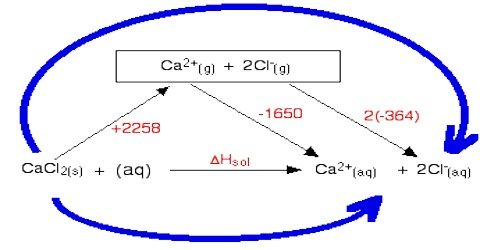# Enthalpy of Solution

Enthalpy of Solution

If some ammonium chloride or glucose is added to water in a test tube the tube becomes cooler. On the other hand if some solid NaOH is added to the test tube the water becomes quite warm. These are examples of a common experience that when solids are dissolved in water heat is either absorbed or evolved. In most uses heat is absorbed so that the solution is cooler than what the solvent was before the addition of the solid. Like other enthalpy changes heat change for the solution process is defined as:

“The enthalpy change when 1 mole of substance is completely dissolved in water”.

Two examples are given below:

Na+ OH (s) + H2O (l) → Na+ (aq) + OH (aq);   ∆H = – 40 kJ mol-1

NH4+ NO3 (s) + H2O (l) → NH4+ (aq) + NO3 (aq);   ∆H = + 26 kJ mol-1

Heat of solution, however, depends on the concentration of the resulting solution. For example, the heat of solution of 1.0 mole of NaOH in 5 moles of water and in 200 moles of water are respectively – 37.8 and – 42.3 kJ. The heat of solution is, therefore, more correctly defined as-

“The change in enthalpy when one mole of a substance a dissolved in a specified quantity of solvent”.

The enthalpy change in this case is termed as integral heat of solution. Another enthalpy term used is differential enthalpy of solution. The distinction between the two quantities can be shown by the following example.

Suppose we make up a solution of 5 moles of KNO3 in 1000 g of water. This can be carried out in two ways. In the first process 5 moles of the salt is added to 1000 g of water and the heat change is measured. If we divide the heat change by 5 we obtain the heat change for solution of 1.0 mole of the salt in 1000 g of water. This quantity is named the integral heat of solution. In the second process 1.0 mole of the salt is added to 1000 g of water and the heat change is measured. Next another mole of the salt is added to this solution and the heat change is measured. In the third step another mole of the salt is added to the solution just prepared and the heat measured. This is continued until the remaining 2 moles are added, one mole at a time and the heat change measured after each addition of one mole. The sum of the heat changes of the 5 steps in the second process would be the same as for the first process, as the initial and final states are the same in both the cases. The 5 heat changes for the 5 steps would, however, be different, if the number of steps is very large then the heat change for each step is called the differential heat of solution.

The differential heat of solution is a partial molar quantity. Hence,

“When one mole of a substance is dissolved in a specified quantity of solvent in a large number of steps the enthalpy change per mole of solid for each step is called the differential heat of solution”.